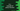# C++ puts() function explanation with examples## puts() function in C++ :

In C++, input/output operations can be performed by using C standard libraries. These are defined inside the cstdio.h header file in C++. Full form of cstdio is C Standard Input and Output library.

In this tutorial, we will learn how to use the puts() function in C++ and its use cases.

## Syntax of puts() :

The puts() function is defined as below :

``````int puts ( const char * str );
``````

In simple words, you can use this function to print a string to the user. It takes one argument str, which is a C string pointer. It writes this str to stdout.

Note that it adds one newline character ‘\n’ after writing the string. This function is defined in cstdio.h file and we need to import it at the beginning of the program.

## Return value :

On success, it returns one non-negative value and on error, it returns EOF.

### Example program :

Let’s take a look at the below example :

``````#include <cstdio>

int main()
{
char str[] = "Hello World !!";

puts(str);
puts(str);

return 0;
}
``````

It prints the below output :

``````Hello World !!
Hello World !!
``````

I have mentioned before that one newline character is automatically added by puts function. This is the reason the second string is printed on a new line.

Try the above example on your machine and drop one comment below if you have any queries.